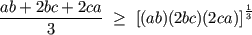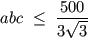1. I worked out this problem today, and thought it was quite neat, so I posted it for anyone else to try:

An open-topped box, has dimensions a, b, (base) and c (height). If the surface area of the box is 100m<sup>2</sup> (outside only), find the largest volume of the box. The faces of the box are 2D (0 thickness).

It probably won't be a hard question for some of you but I thought it was quite a nice little application of what I'm learning.2.

3. One method is to use calculus. Another method is the following:

We have ab + 2bc + 2ca = 100.

By the AM–GM inequality,which on simplification becomesSo the maximum volume is 500*⁄*3√3*m<sup>3</sup>, attained when ab = 2bc = 2ca = 100*⁄*3, i.e. when a = b = 10*⁄*√3*m, c = 5*⁄*√3*m.

This is a typical math-olympiad problem – and problems involving AM–GM abound in math olympiads.4. Nice, I didn't know about that method, but it's the right answer anyway. I just used the calculus method which I though was quite nice...

Surface area = 100 = ab+2ac+2bc

Then get volume as a function of only a and b, V(a,b):

c=(100-ab) / 2(a+b)

Volume=abc= ab(100-ab) / 2(a+b)

Then you can find a maximum point by using ∇f=0

∇V(a,b) = <∂V/∂a, ∂V,∂b>

using the quotient rule:

V= ab(100-ab) / 2(a+b) = u/v

∂V/∂a = [v∂u/∂a - u∂v/∂a] / v<sup>2</sup>
=[(2a+2b)(100-2ab<sup>2</sup>)-2(100ab-a<sup>2</sup>b<sup>2</sup>)] / (2a+2b)<sup>2</sup>

Which simplifies to:

∂V/∂a = [200b<sup>2</sup>-2a<sup>2</sup>b<sup>2</sup>-4ab<sup>3</sup>] / 4(a+b)<sup>2</sup>

Then a similar thing for ∂V/∂b gives:

∂V/∂b = [200a<sup>2</sup>-2a<sup>2</sup>b<sup>2</sup>-4a<sup>3</sup>b] / 4(a+b)<sup>2</sup>

so for ∇V=0,

[200b<sup>2</sup>-2a<sup>2</sup>b<sup>2</sup>-4ab<sup>3</sup>] / 4(a+b)<sup>2</sup>=0

[200a<sup>2</sup>-2a<sup>2</sup>b<sup>2</sup>-4a<sup>3</sup>b] / 4(a+b)<sup>2</sup>=0

Thats only true if the numerator is 0, so:

200b<sup>2</sup>-2a<sup>2</sup>b<sup>2</sup>-4ab<sup>3</sup>=0 (1)
200a<sup>2</sup>-2a<sup>2</sup>b<sup>2</sup>-4a<sup>3</sup>b=0 (2)

a and b are non-zero, since if they were 0, then volume would be zero, so solving simultaneously:

(1) / 2b<sup>2</sup> --> 0=100-a<sup>2</sup>-2ab
(2) / 2a<sup>2</sup> --> 0=100-b<sup>2</sup>-2ab

100-a<sup>2</sup>=100-b<sup>2</sup>
a=b for positive values.

So then 0=100-a<sup>2</sup>-2a<sup>2</sup>,
3a<sup>2</sup>=100

a=√(100/3) = (10√3)/3 = b

c= 100-ab / 2(a+b) = 100- [10<sup>2</sup>*3 / 9] / [40√3 / 3]
= (100 / [40√3 /3]) - (100/3 / [40√3 /3])
=(300 / 40√3) - (100 / 40√3)
=200 /40√3 = 5/√3 = 5√3/3

Then V=abc = (10√3/3)<sup>2</sup>(5√3/3) = (100/3)*(5√3/3)

= 500√3/9 m<sup>2</sup>

It's kind of tedious, especially now I've seen your method.5. Until recently, I would have tackled the problem using calculus as well. It was only recently that I learned to harness the power of the AM–GM inequality.6. Lol, i'm having a little read of it now, I can't understand why it's true, but i'm having a little look on the wiki article. It seems theres a few different proofs for it.7. The proof by induction on the wiki article should be the easiest one of all to follow.Bookmarks
 Posting Permissions
 You may not post new threads You may not post replies You may not post attachments You may not edit your posts   BB code is On Smilies are On [IMG] code is On [VIDEO] code is On HTML code is Off Trackbacks are Off Pingbacks are Off Refbacks are On Terms of Use Agreement